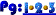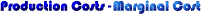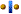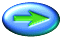Marginal cost is the added cost of increasing output by a small amount. Suppose a firm manufactures oak bookcases. The marginal cost of an additional bookcase might include the costs of the wood, glue, hardware (if any), labor time, and finish applied. All of these are variable inputs since the firm would need more of them in order to produce another bookcase and so make up the marginal cost of the added case.

How do marginal costs change as output increases in the short run? The answer lies in the law of diminishing returns (see the section on Diminishing Returns). We saw that output rose at a decreasing rate in the short run due to the fact that some inputs are fixed in this time frame. This means that marginal cost must be rising. A numerical computation may help clarify this...